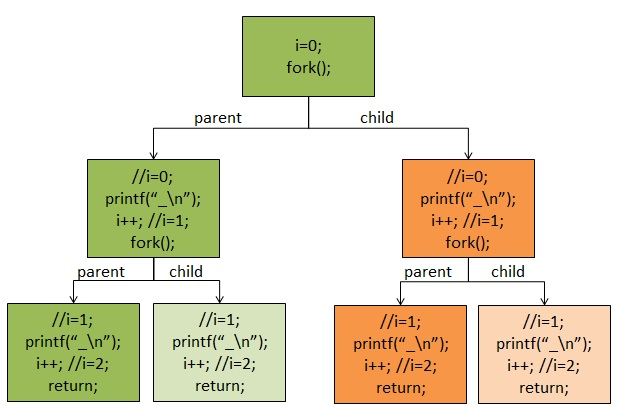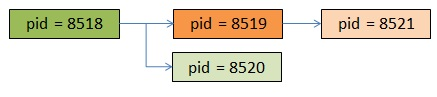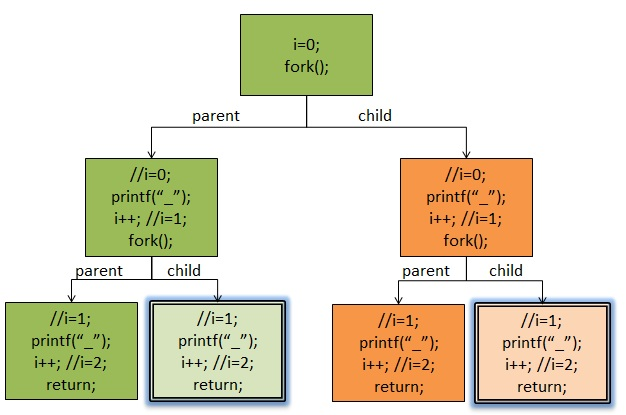# fork对行缓冲区的影响

``````#include <stdio.h>
#include <sys/types.h>
#include <unistd.h>

int main(void)
{
int i;
for(i=0; i<2; i++){
fork();
printf("-");
}

wait(NULL);
wait(NULL);

return 0;
}
``````

fork()系统调用是Unix下以自身进程创建子进程的系统调用，一次调用，两次返回，如果返回是0，则是子进程，如果返回值>0，则是父进程（返回值是子进程的pid），这是众为周知的。

``````printf("-");
fflush(stdout);
``````

``````#include <stdio.h>
#include <sys/types.h>
#include <unistd.h>
int main(void)
{
int i;
for(i=0; i<2; i++){
fork(); //注意：下面的printf有“\n”
printf("ppid=%d, pid=%d, i=%d \n", getppid(), getpid(), i);
}
sleep(10); //让进程停留十秒，这样我们可以用pstree查看一下进程树
return 0;
}
``````

``````ppid=8858, pid=8518, i=0
ppid=8858, pid=8518, i=1
ppid=8518, pid=8519, i=0
ppid=8518, pid=8519, i=1
ppid=8518, pid=8520, i=1
ppid=8519, pid=8521, i=1
``````

\$ pstree -p | grep fork |-bash(8858)-+-fork(8518)-+-fork(8519)—fork(8521) | | `-fork(8520)（全文完）